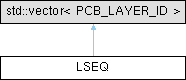LSEQ Class Reference

LSEQ is a sequence (and therefore also a set) of PCB_LAYER_IDs. More...

`#include <layer_ids.h>`

Inheritance diagram for LSEQ:## Public Member Functions

LSEQ ()

template<class InputIterator >
LSEQ (InputIterator aStart, InputIterator aEnd)

void Rewind ()

void operator++ ()

void operator++ (int)

operator bool ()

PCB_LAYER_ID operator* () const

unsigned m_index

## Detailed Description

LSEQ is a sequence (and therefore also a set) of PCB_LAYER_IDs.

A sequence provides a certain order.

It can also be used as an iterator: ` `

`````` for( LSEQ cu_stack = aSet.CuStack();  cu_stack;  ++cu_stack )
{
layer_id = *cu_stack;
:
things to do with layer_id;
}
``````

` `

Definition at line 492 of file layer_ids.h.

## ◆ LSEQ() [1/2]

 LSEQ::LSEQ ( )
inline

Definition at line 498 of file layer_ids.h.

498 :
499 m_index( 0 )
500 {}
unsigned m_index
Definition: layer_ids.h:494

## ◆ LSEQ() [2/2]

template<class InputIterator >
 LSEQ::LSEQ ( InputIterator aStart, InputIterator aEnd )
inline

Definition at line 503 of file layer_ids.h.

503 :
504 BASE_SEQ( aStart, aEnd ), m_index( 0 )
505 {}
std::vector< PCB_LAYER_ID > BASE_SEQ
A sequence of layers, a sequence provides a certain order.
Definition: layer_ids.h:473

## ◆ operator bool()

 LSEQ::operator bool ( )
inline

Definition at line 513 of file layer_ids.h.

513{ return m_index < size(); }

References m_index.

## ◆ operator*()

 PCB_LAYER_ID LSEQ::operator* ( ) const
inline

Definition at line 515 of file layer_ids.h.

516 {
517 return at( m_index ); // throws std::out_of_range
518 }

References m_index.

## ◆ operator++() [1/2]

 void LSEQ::operator++ ( )
inline

Definition at line 509 of file layer_ids.h.

509{ ++m_index; } // returns nothing, used in simple statements only.

References m_index.

## ◆ operator++() [2/2]

 void LSEQ::operator++ ( int )
inline

Definition at line 511 of file layer_ids.h.

511{ ++m_index; }

References m_index.

## ◆ Rewind()

 void LSEQ::Rewind ( )
inline

Definition at line 507 of file layer_ids.h.

507{ m_index = 0; }

References m_index.

## ◆ m_index

 unsigned LSEQ::m_index
private

Definition at line 494 of file layer_ids.h.

Referenced by operator bool(), operator*(), operator++(), and Rewind().

The documentation for this class was generated from the following file: